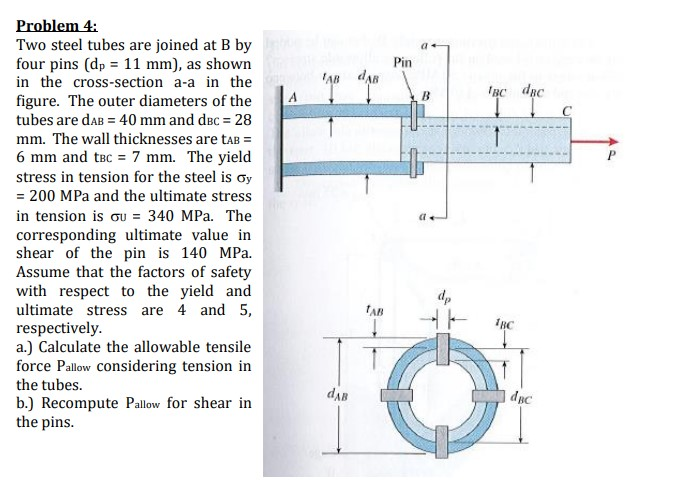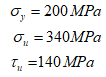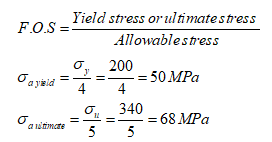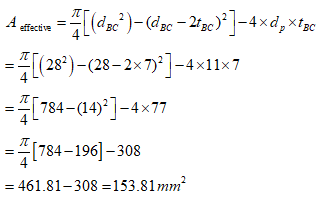# Problem 4:Two steel tubes are joined at B byfour pins (dp = 11 mm), as shownin the cross-section a-a in thefigure. The outer diameters of thePintubes are daB = 40 mm and dec = 28mm. The wall thicknesses are taB =6 mm and tBc = 7 mm. The yieldstress in tension for the steel is oy= 200 MPa and the ultimate stressin tension is ou = 340 MPa. Thecorresponding ultimate value inshear of the pin is 140 MPa.Assume that the factors of safetywith respect to the yield andultimate stress are 4 and 5,respectively.a.) Calculate the allowable tensileforce Pallow Considering tension indpTAB1всthe tubes.dABdpcb.) Recompute Pallow for shear inthe pins.

Question
58 views

Two steel tubes are joined at B by
four pins (dp = 11 mm), as shown
in the cross-section a-a in the
figure. The outer diameters of the
tubes are dAB = 40 mm and dBC = 28
mm. The wall thicknesses are tAB =
6 mm and tBC = 7 mm. The yield
stress in tension for the steel is y
= 200 MPa and the ultimate stress
in tension is U = 340 MPa. The
corresponding ultimate value in
shear of the pin is 140 MPa.
Assume that the factors of safety
with respect to the yield and
ultimate stress are 4 and 5,
respectively.
a.) Calculate the allowable tensile
force Pallow considering tension in
the tubes.
b.) Recompute Pallow for shear in
the pins.help_outlineImage TranscriptioncloseProblem 4: Two steel tubes are joined at B by four pins (dp = 11 mm), as shown in the cross-section a-a in the figure. The outer diameters of the Pin tubes are daB = 40 mm and dec = 28 mm. The wall thicknesses are taB = 6 mm and tBc = 7 mm. The yield stress in tension for the steel is oy = 200 MPa and the ultimate stress in tension is ou = 340 MPa. The corresponding ultimate value in shear of the pin is 140 MPa. Assume that the factors of safety with respect to the yield and ultimate stress are 4 and 5, respectively. a.) Calculate the allowable tensile force Pallow Considering tension in dp TAB 1вс the tubes. dAB dpc b.) Recompute Pallow for shear in the pins. fullscreen
check_circle

Step 1

given:

the diameter of pin, dp = 11 mm

the outer diameter of tubes dAB =40 mm

the outer diameter of tube dBC= 28 mm

the wall thicknes tAB = 6 mm

the wall thickness tBC = 7 mm

The yield strength ,ultimate tensile strength & ultimate shear strength is given as:The factor of safety for ultimate strength = 5

The factor of safety for yield  strength = 4

Step 2

(a)

First, we calculate the allowable stress in yield and ultimate strength criteria.

Factor of safety is defined as:Step 3

Now we calculate the effective area of the tube as across a-a given in problem.

Aeffective = the effective area of the tube for calculation of tensile noramal stress....

### Want to see the full answer?

See Solution

#### Want to see this answer and more?

Solutions are written by subject experts who are available 24/7. Questions are typically answered within 1 hour.*

See Solution
*Response times may vary by subject and question.
Tagged in